# QlikView App Dev

Discussion Board for collaboration related to QlikView App Development.

Announcements
Support Cases coming to Qlik Community Oct. 4! Start chats, open cases, explore resources: READ DETAILS
cancel
Showing results for
Did you mean: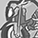Specialist

## Color is not shown correctly!!

Hello,

I have attached a samplpe of application. In that i am using expression to color the values above and below threshhold as RED.

And in between threshhold as BLACK.

=if(Sum(Price) > (Avg(Price) + Avg(Price)*0.02) , RGB(255,0,0),

if(Sum(Price) <  (Avg(Price) - Avg(Price)*0.02) , RGB(255,0,0),

if(Sum(Price) >= ((Avg(Price) - Avg(Price)*0.02) and (Sum(Price) <= (Avg(Price) + Avg(Price)*0.02) , RGB(0,0,0),

)))

But same is not happening . Please see...

1 Solution

Accepted SolutionsMaster III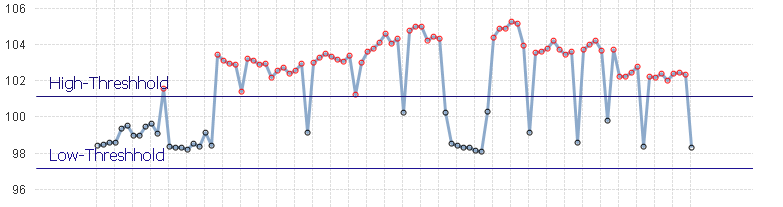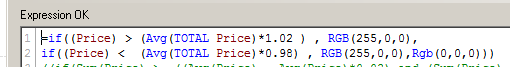17 Replies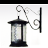Specialist II

Hello Nikhil,

RGB is working well. I think that the problem is that the condition

if(Sum(Price) >= ((Avg(Price) - Avg(Price)*0.02) and (Sum(Price) <= (Avg(Price) + Avg(Price)*0.02)

is never TRUE and that is the reason why it does not show black.

Hth.MVP

Hi,

Try this

=if(Sum(Price) > (Avg(Price) + Avg(Price)*0.02) , RGB(255,0,0),

if(Sum(Price) <  (Avg(Price) - Avg(Price)*0.02) , RGB(255,0,0), RGB(0,0,0),

))

Remove this condition

if(Sum(Price) >= ((Avg(Price) - Avg(Price)*0.02) and (Sum(Price) <= (Avg(Price) + Avg(Price)*0.02),

Regards

Great dreamer's dreams never fulfilled, they are always transcended.Specialist
Author

Hey,

it is also not working.

What should i do ??

ThanksMaster III

Hi NiKhil,

I correct espression [- ',' + 2')'], but I don't know if it work well. I see only red.

RegardsSpecialist
Author

I cannot open your qvw.Can you tell me here.

ThanxMaster III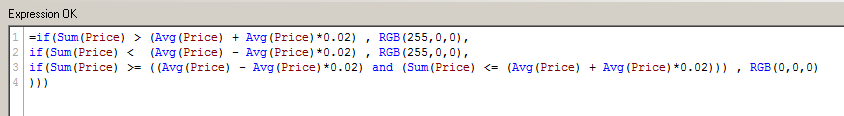Specialist

Yes,

the example of Antonio works well.

=if((Sum(Price) > (Avg(Price) + Avg(Price)*0.02)) , RGB(10,410,0), if(Sum(Price) <  (Avg(Price) - Avg(Price)*0.02) , RGB(25,80,100),

if(Sum(Price) >= (Avg(Price) - Avg(Price)*0.02) and Sum(Price) >= (Avg(Price) + Avg(Price)*0.02), RGB(0,255,80)

)))

I changed the values of RGB to demostrate it

Regards

JAGMVP

Hi,

There is bracket missing in your expression.

You can correct your expression by giving proper bracket closing

Try Like

=if(Sum(Price) > (Avg(Price) + Avg(Price)*0.02) , RGB(255,0,0),

if(Sum(Price) < (Avg(Price) - Avg(Price)*0.02) , RGB(255,0,0),

if(Sum(Price) >= ((Avg(Price) - Avg(Price)*0.02)) and (Sum(Price) <= (Avg(Price) + Avg(Price)*0.02)) , RGB(0,0,0),

)))

or modify it like

=if(Sum(Price) >= ((Avg(Price) - Avg(Price)*0.02)) and (Sum(Price) <= (Avg(Price) + Avg(Price)*0.02)),RGB(0,0,0),
if(Sum(Price) > (Avg(Price) + Avg(Price)*0.02),RGB(255,0,0),
if(Sum(Price) <  (Avg(Price) - Avg(Price)*0.02) , RGB(255,255,255),
)))

or

you can try this also

=if(Sum(Price) > (Avg(Price) + Avg(Price)*0.02) , RGB(255,0,0),
if(Sum(Price) <  (Avg(Price) - Avg(Price)*0.02) , RGB(255,255,255), RGB(0,0,0)
))

and check your logic which you are comparing in if. I am not sure that it is working proper.

Kind Regards

Great dreamer's dreams never fulfilled, they are always transcended.Specialist
Author

HEy,

It is still not working. I mean ,  I want the values above High threshhold and below Low threshhold as RED and between High threshhold and LowThreshhold as BLACK. PRice is my field.

How to do this ???Community Browser